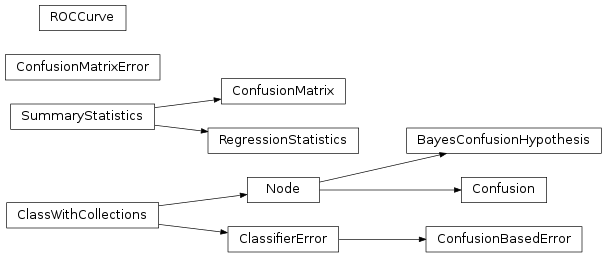# mvpa2.clfs.transerror¶

Utility class to compute the transfer error of classifiers.Functions

 `Doi`(\*args, \*\*kwargs) Perform no good and no bad `auc_error`(predicted, target) Computes the area under the ROC for the given the target and predicted to make the prediction. `auto_null_dist`(dist) Cheater for human beings – wraps `dist` if needed with some `ceil`(x) Return the ceiling of x as a float. `chisquare`(obs[, exp]) Compute the chisquare value of a contingency table with arbitrary dimensions. `corr_error`(predicted, target) Computes the correlation between the target and the predicted values. `corr_error_prob`(predicted, target) Computes p-value of correlation between the target and the predicted values. `enhanced_doc_string`(item, \*args, \*\*kwargs) Generate enhanced doc strings for various items. `friedmanchisquare`(\*args) Computes the Friedman test for repeated measurements `linregress`(x[, y]) Calculate a linear least-squares regression for two sets of measurements. `log10`(x) Return the base 10 logarithm of x. `mean_mismatch_error`(predicted, target) Computes the percentage of mismatches between some target and some predicted values. `mean_power_fx`(data) Returns mean power `nanmean`(a[, axis, dtype, out, keepdims]) Compute the arithmetic mean along the specified axis, ignoring NaNs. `relative_rms_error`(predicted, target) Ratio between RMSE and root mean power of target output. `rms_error`(predicted, target) Computes the root mean squared error of some target and some predicted values. `root_mean_power_fx`(data) Returns root mean power `table2string`(table[, out]) Given list of lists figure out their common widths and print to out

Classes

 `BayesConfusionHypothesis`([alpha, ...]) Bayesian hypothesis testing on confusion matrices. `ClassWithCollections`([descr]) Base class for objects which contain any known collection `ClassifierError`(clf[, labels, train]) Compute (or return) some error of a (trained) classifier on a dataset. `Collectable`([value, name, doc]) Collection element. `ConditionalAttribute`([enabled]) Simple container intended to conditionally store the value `Confusion`([attr, labels, add_confusion_obj]) Compute a confusion matrix from predictions and targets (Node interface) `ConfusionBasedError`(clf[, labels, ...]) For a given classifier report an error based on internally computed error measure (given by some `ConfusionMatrix` stored in some conditional attribute of `Classifier`). `ConfusionMatrix`([labels, labels_map]) Class to contain information and display confusion matrix. `ConfusionMatrixError`([labels]) Compute confusion matrix as an “error function” `Dataset`(samples[, sa, fa, a]) Generic storage class for datasets with multiple attributes. `Node`([space, pass_attr, postproc]) Common processing object. `ROCCurve`(labels[, sets]) Generic class for ROC curve computation and plotting `RegressionStatistics`(\*\*kwargs) Class to contain information and display on regression results. `StringIO`([buf]) class StringIO([buffer]) `SummaryStatistics`([targets, predictions, ...]) Basic class to collect targets/predictions and report summary statistics

Exceptions

 `BayesConfusionHypothesis`([alpha, ...]) Bayesian hypothesis testing on confusion matrices. `ClassWithCollections`([descr]) Base class for objects which contain any known collection `ClassifierError`(clf[, labels, train]) Compute (or return) some error of a (trained) classifier on a dataset. `Collectable`([value, name, doc]) Collection element. `ConditionalAttribute`([enabled]) Simple container intended to conditionally store the value `Confusion`([attr, labels, add_confusion_obj]) Compute a confusion matrix from predictions and targets (Node interface) `ConfusionBasedError`(clf[, labels, ...]) For a given classifier report an error based on internally computed error measure (given by some `ConfusionMatrix` stored in some conditional attribute of `Classifier`). `ConfusionMatrix`([labels, labels_map]) Class to contain information and display confusion matrix. `ConfusionMatrixError`([labels]) Compute confusion matrix as an “error function” `Dataset`(samples[, sa, fa, a]) Generic storage class for datasets with multiple attributes. `Node`([space, pass_attr, postproc]) Common processing object. `ROCCurve`(labels[, sets]) Generic class for ROC curve computation and plotting `RegressionStatistics`(\*\*kwargs) Class to contain information and display on regression results. `StringIO`([buf]) class StringIO([buffer]) `SummaryStatistics`([targets, predictions, ...]) Basic class to collect targets/predictions and report summary statistics